﻿ Vibration Control of an Electromechanical Model with Time-Dependent Magnetic Field

### Vibration Control of an Electromechanical Model with Time-Dependent Magnetic Field

Usama H. Hegazy, Jihad Y. Abu Ful

## Vibration Control of an Electromechanical Model with Time-Dependent Magnetic Field

Usama H. Hegazy1,, Jihad Y. Abu Ful1

1Department of Mathematics, Faculty of Science, Al-Azhar University, Gaza, Palestine

### Abstract

This paper presents a study of the nonlinear response of the electromechanical (seismograph) system under parametric excitations in the mechanical and electrical parts with periodically time-varying magnetic field. The case of subharmonic (parametric) resonance is considered and examined. Approximated solutions are sought applying the method of multiple scales. Numerical simulations are carried out to illustrate the steady-state response and the stability of the solutions using the frequency response function and time series solution.

• Usama H. Hegazy, Jihad Y. Abu Ful. Vibration Control of an Electromechanical Model with Time-Dependent Magnetic Field. Journal of Mechanical Design and Vibration. Vol. 4, No. 1, 2016, pp 1-9. http://pubs.sciepub.com/jmdv/4/1/1
• Hegazy, Usama H., and Jihad Y. Abu Ful. "Vibration Control of an Electromechanical Model with Time-Dependent Magnetic Field." Journal of Mechanical Design and Vibration 4.1 (2016): 1-9.
• Hegazy, U. H. , & Ful, J. Y. A. (2016). Vibration Control of an Electromechanical Model with Time-Dependent Magnetic Field. Journal of Mechanical Design and Vibration, 4(1), 1-9.
• Hegazy, Usama H., and Jihad Y. Abu Ful. "Vibration Control of an Electromechanical Model with Time-Dependent Magnetic Field." Journal of Mechanical Design and Vibration 4, no. 1 (2016): 1-9.

 Import into BibTeX Import into EndNote Import into RefMan Import into RefWorks

123
Prev Next

### 1. Introduction

Considerable effort has been devoted to the study of nonlinear oscillations and chaotic motion of some coupled oscillators in several scientific fields [1, 2]. The nonlinear oscillations and chaotic motions in a controlled electromechanical seismograph system with time-varying stiffness is investigated . The stiffness in the seismograph is considered as the time varying in a periodic form. The method of multiple time scale perturbation technique is used to solve the nonlinear differential equations describing the controlled system up to second order of accuracy, where different active controllers are applied. The dynamics and synchronization of two coupled electromechanical devices and effects of higher nonlinearity were investigated , where harmonic balance is utilized to derive the amplitude equations in the general case. The averaging method is used to study the oscillatory state of a self-sustained electromechanical system . The effects of the nonlinear coupling, detuning parameter and chaotic behavior were investigated. Chaos control, bifurcation diagrams of the electromechanical seismograph system were studied . The amplitudes of the fifth subharmonic and superharmonic vibrations states of the system were found using the multiple scales method. The appropriate coupling parameter of an electromechanical damping device with magnetic coupling was found analytically and numerically . The problem of suppressing the vibrations of a hinged-hinged flexible beam when subjected to external harmonic and parametric excitations is considered and studied. The multiple scale perturbation method is applied to obtain a first-order approximate solution. The equilibrium curves for various controller parameters are plotted. The stability of the steady state solution is investigated using frequency-response equations. The approximate solution was numerically verified. It is found that all predictions from analytical solutions were in good agreement with the numerical simulations . The bifurcation structure of the model was analyzed and the effects of the coupling parameter on the bifurcation structure was studied. An extended Duffing-Van der Pol oscillator was considered and the method of multiple scales is utilized to obtain the approximate solution both in the case of non-resonant and resonant states . Moreover, the Melinkov theorem is used to investigate the chaotic behavior of the system. A particular case of the micro-elctro-mechanical system (MEMS) model is introduced by including a time-varying stiffness, and the motion of the model is studied using the phase portrait, time series, and Poincare map . It is shown from the phase portrait, and Poincare map that there exist different motions in the MEMS resonator system under certain conditions. Melnikov’s method has been employed to define the regions of parameter space where homoclinic and heteroclinic chaos can occur. Numerical simulations are performed to study the system’s behavior for various parameter sets and to verify the result from Melnikov’s method. in the self-sustained electromechanical system with multiple functions is considered, and he averaging and the harmonic balance methods were used to analyze the amplitudes of the oscillatory states in the autonomous and nonautonomous cases, respectively . Different bifurcation structures, stability chart and the variation of the Lyapunov exponent were obtained. The dynamics and chaos control of the self-sustained electromechanical device with and without discontinuity were studied . The effects of the amplitude of the parametric modulation and some coefficients along with the transition to chaotic behavior were studied.

In this paper, the steady-state response of the seismograph model with time-dependent magnetic field is investigated for various system parameters under subharmonic resonance condition. The stability of the numerical solution is studied using the frequency response function method. Three active control laws have been applied and their performance is investigated. Numerical integration using Runge-Kutta fourth order is performed to verify analytical results obtained by the method of multiple scales perturbation technique.

### 2. Approximate Perturbation Solution

The considered seismograph system will be subjected to parametric excitations with periodically time-varying magnetic field. The modified governing equations of motion are given by ,(1)(2)

where, the coefficients are the same as those defined in .

The method of multiple scales is used to obtain approximate solutions of the nonlinear equations (1) and (2). Assuming x and q in the form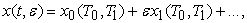(3)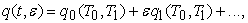(4)

where T0 = t is the fast time scale associated with changes occurring at the frequencies ω, ω1,2 and Ω, and T1 = εt is the slow time scale associated with modulations in the amplitudes and phases caused by the nonlinearity, damping, and resonances.

In terms of T0 and T1, the time derivatives become: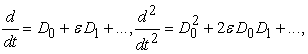where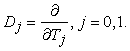Then substituting equations (3) and (4) and the time derivatives into equations (1) and (2) and comparing the coefficients of the same powers of ε, we obtain:(5)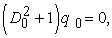(6)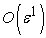: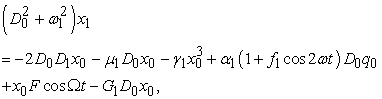(7)(8)

The solutions of (5) and (6) can be expressed as:where A0, B0 are complex functions in T1 .

Substituting for x0 and q0 into equations (7) and (8), then the general solutions of equations (1) and (2) can be expressed in the following simplified form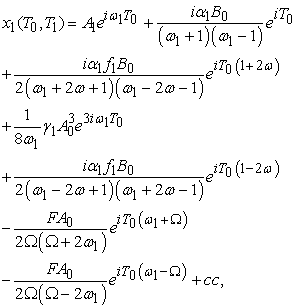(9)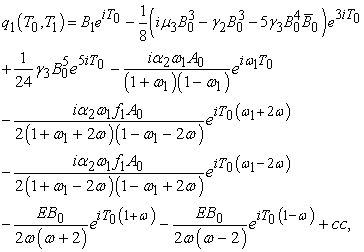(10)

### 3. Subharmonic Resonance Analysis

In this section, the principal parametric resonance (subharmonic) which occurs when the frequency of the excitation is close to twice that of the natural frequencies of the system, that is (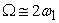) and (), in the presence of one-to-one internal resonance (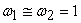). The previous resonant relations can be expressed as follows(11)

Eliminating the secular terms from equations (9) and (10), the solvability condition yields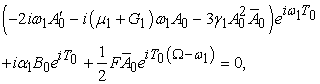(12)(13)

Substituting equation (11) into equations (12) and (13) then dividing by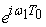and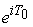, respectively, we get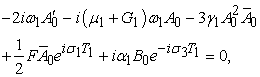(14)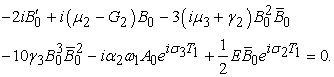(15)

Substituting the polar forms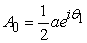,, whereand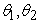are the steady-state amplitudes and the phases of motions, respectively, and simplifying we obtain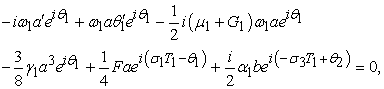(16)(17)

Dividing (16) byand (17) by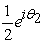yield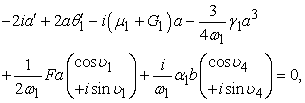(18)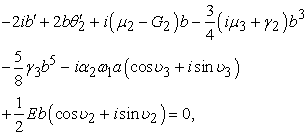(19)

where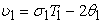,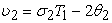,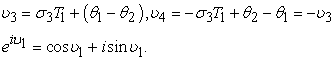Separating real and imaginary parts, gives the governing equations of the amplitudes a, b and phases υi(20)(21)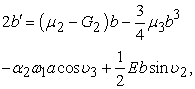(22)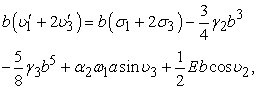(23)

The steady-state solutions correspond to constant a, b, υi, (i =1,…,4); that is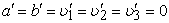. Then equations (20) – (23), which describe the modulation of the amplitudes and the phases for the parametric resonance response of the system (1) and (2), become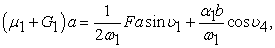(24)(25)(26)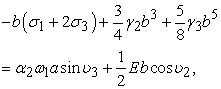(27)

Squaring (24-27), adding (24) and (25), then (26) and (27) yields the following frequency response equations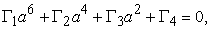(28)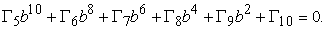(29)

The coefficients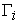, for i=1,2,…,10 are defined in Appendix C.

### 4. Numerical Results

In this section, the steady-state response of the system is investigated for various system parameters under the subharmonic resonance condition. The stability of the numerical solution is studied using the frequency response function method.

The frequency response Equations (28) and (29) which are nonlinear algebraic equations in the amplitude a and b respectively are solved. The results are shown in Figure 1 as the amplitude a of the mechanical part against the detuning parameter σ2, and in Figure 2 as the amplitude b of the electrical part against the detuning parameter σ1 for different values of the system parameters.

Figures 1(a), (c) , (d) and (h) show that the steady-state amplitude a decreases as each of the natural frequency ω1, the damping coefficient μ1, the nonlinear coefficient γ1 and the gain G1 increase. It can also be seen that the branches of the response curves converge to each other and the region of unstable solutions get smaller. But in Figures 1(e) and (g) the steady- state amplitude a increases as each of the second mode amplitude b and the mechanical excitation amplitude F increase. Figure 1(f) indicates that the curves are shifted as the detuning parameter σ3 varies. The effect of varying the coupling coefficient α1 on the frequency response is trivial, as shown in Figures 1(b).Download asVeiw figureFigures index
Figure 1. Frequency response curves of the mechanical part at subharmonic resonance casewith linear velocity feedback when: F=3.0, γ1=2.0, ω1=1.0, σ3=0.01, μ1=0.1, α1=2.5, b=0.01, G1=0.1

It is shown from Figure 2(g) that the branches of frequency response curve diverge and the unstable region increases as the first mode amplitude a increase. This would explain that there might be a transfer of energy from the first mode to the second mode through the coupling parameters and the internal resonance as well. Whereas Figure 2(i), indicates that the steady-state amplitude b increases as the electrical amplitude E increase. Figures 2(a) and (b) show that the effects of varying the natural frequency ω1 and the coupling coefficient α2 are trivial. But from Figures 2(c), (d), (e), (f) and (j) we can see that the steady-state amplitude b increases as each of the linear damping coefficient μ2, the nonlinear damping coefficient μ3, the nonlinear coefficient γ2, the nonlinear coefficient γ3 and the gain G2 decrease. Figure 2(h) indicates a shifting effect to the left and an increase in the amplitude as the detuning parameter σ3 increases.Download asVeiw figureFigures index
Figure 2. Frequency response curves of the electrical part at resonance case with linear velocity feedback, when: E=2.0, γ2=0.07, γ3=0.7, ω1=1.0, σ3=0.01, μ2=0.03, μ3=0.2, α2=0.02, a=0.01, G2=0.1

Figure 3 and Figure 4 show the force response curves of the mechanical part and electrical part, respectively, at resonance case with linear velocity feedback. We can see in Figures 3(a), (c), (d), (f) and (h) that the amplitude of the mechanical part a increases as each of the natural frequency ω1, the linear coefficient µ1, the nonlinear coefficient γ1, the detuning parameters σ3, and the gain G1 decrease. Figure 3(b) shows that as the mechanical force lies in the range 0 < F < 0.4, the amplitude a increases as the coupling coefficient α1 is increased. But if F > 0.4, then any further increase in the coupling coefficient α1 has insignificant effect on the amplitude and may lead to saturation phenomenon. From Figure 3(e) we can see that the first mode amplitude a increases as second mode amplitude b increases.Download asVeiw figureFigures index
Figure 3. Force response curves of the mechanical part at resonance case with linear velocity feedback, when: σ2=0.05, γ1=2.0, ω1=1.0, σ3=0.01, μ1=0.1, α1=2.5, b=0.01, G1=0.1Download asVeiw figureFigures index
Figure 4. Force response curves of the electrical part at resonance case with linear velocity feedback when: σ1=0.05, γ2=0.07, γ3=0.7, ω1=1.0, σ3=0.01, μ2=0.03, μ3=0.2, α2=0.6, a=0.01, G2=0.1

It can be shown from Figures 4(c), (d) and (j) that the electrical excitation amplitude E increases as each of the linear coefficient µ2 , the nonlinear coefficient µ3, and the gain G2 decrease. But it can be seen from Figures 4 (a), (b) and (g) that the electrical excitation amplitude E increases as each of the natural frequency ω1, the coupling coefficient α2 and the first mode amplitude a increase. Moreover, a saturation phenomenon is noticed for the system as these parameters are increased when E > 0.3. Figures 4(e), (f), (h) and (i) show the effects of each of the nonlinear coefficients γ2,3 and the detuning parameters σ3 and σ1.

To verify the analytic predictions, Eqs. (1) and (2) are numerically integrated using a fourth order Runge-Kutta algorithm. A non-resonance system behavior is shown in Figure 5. Different resonance cases are investigated and shown in Figure 6, where the steady-state amplitude x has maximum peak at the subharmonic resonance case () in Figure 6 (d). It is noticed that compared to the nonresonant case, the steady-state amplitude x is increased by 900%, while the steady-state amplitude q has not changed. Thus, it is considered as the worst resonance case of the system behavior.Download asVeiw figureFigures index
Figure 5. Non-resonant time history without control when: Ω=3.0, ω1=2.7, ω=3.5, F=0.05, E=0.05 , γ1=0.04, γ2=0.07, γ3=0.7, f1 =0.1, μ1=0.1, μ2=0.3, μ3=0.2, α1=2.5, α2=0.02Download asVeiw figureFigures index
Figure 6. Time history solution at different resonance cases without control when: Ω=3.0, ω1=2.7, ω=3.5, F=0.05, E=0.05 , γ1=0.04, γ2=0.07, γ3=0.7, f1 =0.1, μ1=0.1, μ2=0.3, μ3=0.2, α1=2.5, α2=0.02Download asVeiw figureFigures index
Figure 7. Subharmonic resonance time history with various control laws, when: Ω=2.0, ω1=1.0, ω=2.0, F=0.05, E=0.05, γ1=0.04, γ2=0.07, γ3=0.7, f1 =0.1, μ1=0.1, μ2=0.3, μ3=0.2, α1=2.5, α2=0.02, G1=G2=0.2

From Figure 7(b) we can see that the best control method is the negative linear velocity feedback. The effect of varying the gains G1 and G2 on the x and q amplitudes are shown in Figures 8(a) – (e).Download asVeiw figureFigures index
Figure 8. Effects of the linear velocity feedback gains G1 and G2 on the subharmonic resonance time solution, when : Ω=2.0, ω1=1.0, ω=2.0, F=0.05, E=0.05, γ1=0.04, γ2=0.07, γ3=0.7, f1 =0.1, μ1=0.1, μ2=0.3, μ3=0.2, α1=2.5, α2=0.02Download asVeiw figureFigures index
Figure 9. Effect of the parametric excitation amplitude f1 at resonance case without control

The effect of parametric excitation f1, the amplitude of time varying magnetic field, is studied at subharmonic resonance case and shown in Figure 9, which represents the time-series solutions (t, x) for the mechanical part , and (t, q) for the electrical part. Considering Figure 9(a) as basic case for comparison, it can be seen from Figure 9 that as the parametric excitation increases, a chaotic motion occurs for the x amplitude and the q amplitude. Figures 9(b) – (e) indicate that a continuous increase in f1 changes the shape of the chaotic motion.

### 5. Conclusions

The response and stability of the system of coupled non-linear differential equations representing the non-linear dynamical two-degree-of-freedom electromechanical system including cubic and quintic nonlinearities subject to parametric excitation forces with time-varying magnetic field are solved and studied. The investigation includes the solutions applying both the perturbation technique and Runge-Kutta numerical method. The stability of the system is investigated applying both the frequency response equation and time series solution. From the study it is concluded that the active controllers are very effective tool in vibration reduction at many different resonance cases. They are very helpful in suppressing the undesired vibration or sometimes eliminate them. The end of the work gives some recommendations regarding the design of such system. Also a comparison with similar published work is reported. In this section, the main conclusions of the system are reported briefly.

1. It is found that the frequency response curves consist of two branches. These curves are bent to right or left for some curves due to the nonlinearity effect (hardening or softening). This leads to multi-valued solutions and hence to a jump phenomenon occurrence, which are typical characteristics of the behavior of nonlinear dynamical system and are an important challenge in the controllers design. These results are in agreement with the results in .

2. The steady-state amplitudes x and q of the mechanical and electrical parts, respectively, of the system are monotonic increasing functions in the mechanical and electrical forces F and E, respectively.

3. The steady-state amplitude x of the mechanical part is

i) a monotonic increasing function in the steady-state amplitude q and the coupling coefficient α1.

ii) a monotonic decreasing function in the linear damping coefficient μ1, the nonlinear coefficient γ1 and the gain G1.

4. The steady-state amplitude q of the electrical part is

i) a monotonic increasing function in the steady-state amplitude x, the natural frequency of the mechanical part ω1 and the coupling coefficient α2.

ii) a monotonic decreasing function in the linear and nonlinear damping coefficients μ1 and μ3, the nonlinear coefficients γ1 and γ3 and the gain G2.

5. The force response curves could detect the behavior of some parameters that show insignificant (trivial) effect in the frequency response curves. It also gives an indication to the values of some parameters at which saturation may occur.

6. The applied parametric forces produce various resonance cases. The resonance case with maximum peak of amplitudes is considered as the worst resonance case. Numerical results show that the worst resonance case under parametric forces occurs at the subharmonic resonance case at which the frequency of the excitation is twice that of the natural frequency of the system.

7. As for the parametric excitations f1 and f2, which are the amplitudes of the time-varying magnetic field. When the magnitude of any excitation is increased a chaotic behavior occurs in both modes of the system. The chaotic motion changes as the parametric excitation changes. Moreover, the shapes of chaotic motions for both modes are different. Whereas in , the parametric excitation, which results from varying the stiffness of the mechanical oscillator periodically, has an effect on the x-amplitude only. Thus, stability and controllability in electromechanical seismograph models with time-varying magnetic field are better than seismograph models with constant or time-varying stiffness.

8. Different control laws were applied to the system under parametric excitation forces. It is noticed that the best control method is the negative velocity feedback. Moreover, the higher the gain (G1 or G2) is, the faster is the approach to the equilibrium solution, which is in agreement with . This control force has also an effect in reducing or eliminating nonlinearity effect in the frequency response curves.

### References

  Xiujing, H. and Qinsheng, B, “Bursting oscillations in Duffing’s equation with slowly damping external forcing,” Commun Nonlinear Sci Numer Simul, 16. 4146-4152. 2011.In article View Article  Kitio Kwuimy, C.A. and Woafo P, “Experimental realization and simulation a self-sustained macro electromechanical system,” Mech Res Commun, 37 (1). 106-110. 2010.In article View Article  Hegazy, U.H., “Dynamics and control of a self-sustained electromechanical seismographs with time varying stiffness,” Meccanica, 44, 355-368. 2009.In article View Article  Mbouna Ngueuteu, G.S., Yamapi, R. and Woafo, P, “Effects of higher nonlinearity on the dynamics and synchronization of two coupled electromechanical devices,” Commun Nonlinear Sci Numer Simul, 13. 1213-1240. 2008.In article View Article  Kitio Kwuimy, C.A. and Woafo, P, “Dynamics of a self-sustained electromechanical system with flexible arm and cubic coupling,” Commun Nonlinear Sci Numer Simul, 12, 1504-1517. 2007.In article View Article  Siewe Siewe, M., Moukam Kakmeni, F.M., Bowong S. and Tchawoua C, “Non-linear response of a self sustained electromechanical seismographs to fifth resonance excitations and chaos control,” Chaos Solitons Fractals, 29. 431-445. 2006.In article View Article  Yamapi, R, “Dynamics of an electromechanical damping device with magnetic coupling,” Commun Nonlinear Sci Numer Simul, 11. 907-921. 2006.In article View Article  Hegazy, U.H, “Single-Mode response and control of a hinged-hinged flexible beam,” Arch Appl Mech, 79. 335-345. 2009.In article View Article  Siewe Siewe, M., Moukam Kakmeni, F.M., Tchawoua C, “Resonant oscillation and homoclinic bifurcation in a Ф6-Van der Pol oscillator,” Chaos Solitons Fractals, 21. 841-853. 2004.In article View Article  Siewe Siewe, M., Hegazy U.H, “Homoclinic bifurcation and chaos control in MEMS resonators,” App Math Modell, 35. 5533-5552. 2011.In article View Article  Yamapi, R., Aziz-Alaoui, M.A, “Vibration analysis and bifurcations in the self-sustained electromechanical system with multiple functions,” Commun Nonlinear Sci Numer Simul, 12. 1534-1549. 2007.In article View Article  Yamapi, R., Bowong, S, “Dynamics and chaos control of the self-sustained electromechanical device with and without discontinuity,” Commun Nonlinear Sci Numer Simul, 11. 355-375. 2006.In article View Article